Question

# proofs based analysis 1. Suppose is differentiable on (a, b) and that there exists MER such...

proofs based analysis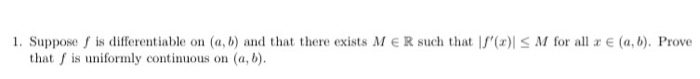1. Suppose is differentiable on (a, b) and that there exists MER such that l'() S M for all x E (a,b). Prove that is uniformly continuous on (a,b).

We need at least 10 more requests to produce the answer.

0 / 10 have requested this problem solution

The more requests, the faster the answer.

All students who have requested the answer will be notified once they are available.

#### Earn Coins

Coins can be redeemed for fabulous gifts.

Similar Homework Help Questions
• ### 3. (a) Suppose f : (a, b) + R is differentiable, and there exists M E...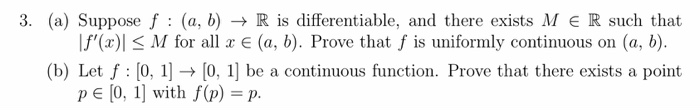3. (a) Suppose f : (a, b) + R is differentiable, and there exists M E R such that If'(x) < M for all x € (a, b). Prove that f is uniformly continuous on (a, b). (b) Let f : [0, 1] → [0, 1] be a continuous function. Prove that there exists a point pe [0, 1] with f(p) = p.

• ### 2. Let f: R R be a continuous function. Suppose that f is differentiable on R\{0} and that there exists an L e R such that lim,of,(z) = L. Prove that f is differentiable at 1-0 with f,(0) = L. (Hint:...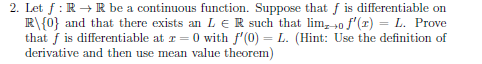2. Let f: R R be a continuous function. Suppose that f is differentiable on R\{0} and that there exists an L e R such that lim,of,(z) = L. Prove that f is differentiable at 1-0 with f,(0) = L. (Hint: Use the definition of derivative and then use mean value theorem) 2. Let f: R R be a continuous function. Suppose that f is differentiable on R\{0} and that there exists an L e R such that lim,of,(z) =...

• ### differentiable function and there exists 0 <A < 1 (6) Suppose that f : R" -> R" is a such that |f'...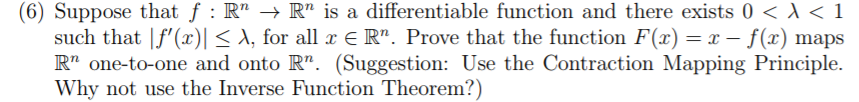differentiable function and there exists 0 <A < 1 (6) Suppose that f : R" -> R" is a such that |f'(x)|< A, for all x E R". Prove that the function F(x)= x - f(x) maps R" one-to-one and onto R". (Suggestion: Use the Contraction Mapping Principle Why not use the Inverse Function Theorem?) differentiable function and there exists 0

• ### 3. Suppose f : [0,) + R is a continuous function and that L limf(x) exists...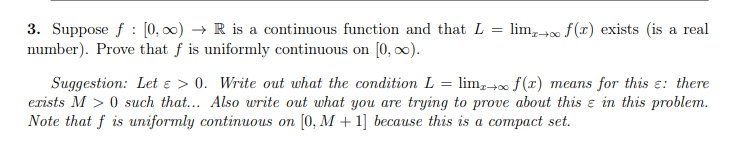3. Suppose f : [0,) + R is a continuous function and that L limf(x) exists is a real number). Prove that f is uniformly continuous on (0,.). Suggestion: Let e > 0. Write out what the condition L = lim,+ f(t) means for this e: there erists M > 0 such that... Also write out what you are trying to prove about this e in this problem. Note that f is uniformly continuous on (0.M +1] because this is...

• ### 4 Suppose f : (0,0) → (0,x), is a differentiable function satisfying f(a +b)-f(a)fb), for all...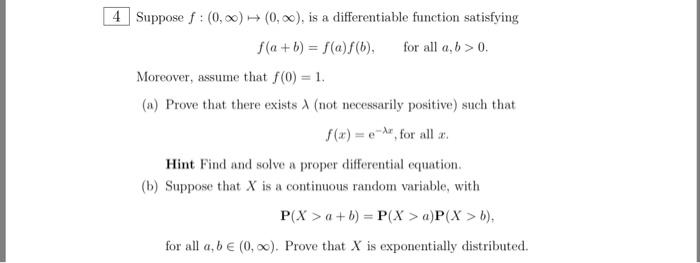4 Suppose f : (0,0) → (0,x), is a differentiable function satisfying f(a +b)-f(a)fb), for all a,b>0 Moreover, assume that f(0)1 (a) Prove that there exists λ (not necessarily positive) such that f(r) = e-Ar, for all r. Hint Find and solve a proper differential equation. (b) Suppose that X is a continuous random variable, with P(X>ab)-P(>a)P(X> b), for all a, b e (0, oo). Prove that X is exponentially distributed

• ### Problem 1. Suppose that f:(a,b) + R is a continuous function and there exists a point...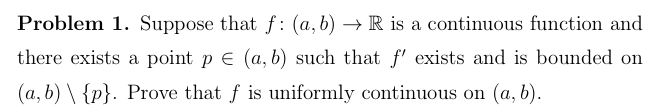Problem 1. Suppose that f:(a,b) + R is a continuous function and there exists a point p e (a, b) such that f' exists and is bounded on (a,b) {p}. Prove that f is uniformly continuous on (a,b).

• ### (a) Suppose f is continuously differentiable on the closed and bounded interval I = [0, 1]....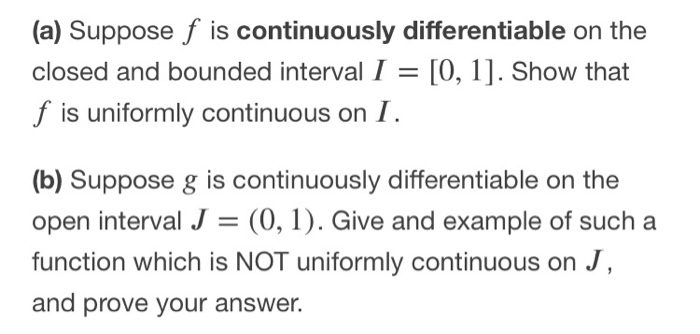(a) Suppose f is continuously differentiable on the closed and bounded interval I = [0, 1]. Show that f is uniformly continuous on I. (b) Suppose g is continuously differentiable on the open interval J = (0,1). Give and example of such a function which is NOT uniformly continuous on J, and prove your answer.

• ### real analysis 1,2,3,4,8please 5.1.5a Thus iff: I→R is differentiable on n E N. is differentiable on / with g&#39...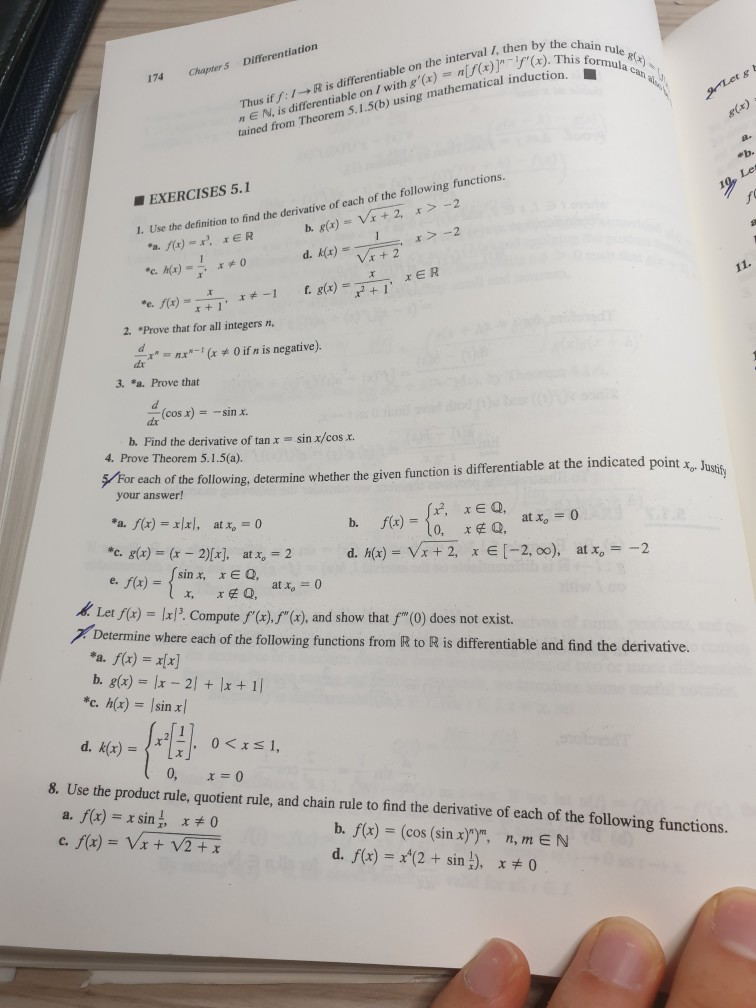real analysis 1,2,3,4,8please 5.1.5a Thus iff: I→R is differentiable on n E N. is differentiable on / with g'(e) ()ain tained from Theorem 5.1.5(b) using mathematical induction, TOu the interal 1i then by the cho 174 Chapter s Differentiation ■ EXERCISES 5.1 the definition to find the derivative of each of the following functions. I. Use r+ 1 2. "Prove that for all integers n, O if n is negative). 3. "a. Prove that (cosx)--sinx. -- b. Find the derivative...

• ### 4. Let {S.} be a sequence of differentiable real-valued functions on (a, b) that converges pointwise...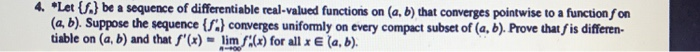4. Let {S.} be a sequence of differentiable real-valued functions on (a, b) that converges pointwise to a function f on (a, b). Suppose the sequence {f) converges uniformly on every compact subset of (a, b). Prove thatf is differen- tiable on (a, b) and that f'(x) = lim f(x) for all x E (a, b). 4. Let {S.} be a sequence of differentiable real-valued functions on (a, b) that converges pointwise to a function f on (a, b). Suppose...

• ### 1(a) Let f : R2 → R b constant M > 0 such that livf(x,y)|| (0.0)-0. Assume that there exists a e continuously differentiable, with Mv/r2 + уг, for all (z. y) E R2 If(x,y)| 〈 M(x2 + y2)· for all (...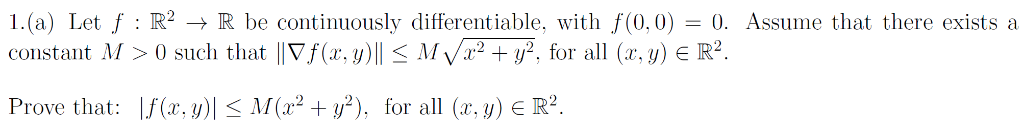1(a) Let f : R2 → R b constant M > 0 such that livf(x,y)|| (0.0)-0. Assume that there exists a e continuously differentiable, with Mv/r2 + уг, for all (z. y) E R2 If(x,y)| 〈 M(x2 + y2)· for all (a·y) E R2 Prove that: 1(a) Let f : R2 → R b constant M > 0 such that livf(x,y)|| (0.0)-0. Assume that there exists a e continuously differentiable, with Mv/r2 + уг, for all (z. y) E R2...